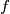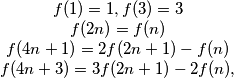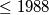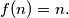### IMO Shortlist 1988 problem 26

Kvaliteta:
Avg: 0,0
Težina:
Avg: 0,0
A function$f$ defined on the positive integers (and taking positive integers values) is given by:$\begin{matrix} f(1) = 1, f(3) = 3 \\ f(2n) = f(n) \\ f(4n + 1) = 2f(2n + 1) - f(n) \\ f(4n + 3) = 3f(2n + 1) - 2f(n)\text{,} \end{matrix}$
for all positive integers$n.$ Determine with proof the number of positive integers$\leq 1988$ for which$f(n) = n.$
Izvor: Međunarodna matematička olimpijada, shortlist 1988